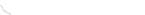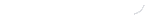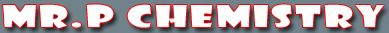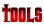activity seriesbond energybond lengthscalculatorcommon namesconversion factors: energyconversion factors: lengthconversion factors: massconversion factors: pressureconversion factors: temp.conversion factors: volumecovalent prefixesdensity solverdiatomic elementse-config. chartelement listgas law formulasKa's of polyprotic acidsKa's of weak acidsKb's of weak basesmetric conversion chartmole conversion chartmolecular geometriesorganic prefixesperiodic tableperiodic table (flash)pH/pOH converterpolyatomic ionspressure converterSI unitssolubility chartsolubility of salts rulessolubility product constantsstoichiometry charttemp. conversiontemp. formulasthermodynamic datavapor pressure of water
Period 5 Homework

Period 5 Homework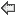September - 2020
Sun Mon Tue Wed Thu Fri Sat
 1
 2
 3
 4
 5
 6
 7
 8
 9
 10
 11
 12
 13
 14
 15
 16
 17
 18
 19
 20
 21
 22
 23
 24
 25
 26
 27
 28
 29
 30
Displaying 1 to 5 of 5 records.
date assigned
assignment
worksheet
Thursday, 9.17.20
Here is a link to today's powerpoint.
Monday, 9.14.20
Here is a link to today's powerpoint.
Thursday, 9.10.20
Complete the following Chapter 1 on-line quizzes: convert to celsius quiz, convert to kelvin quiz, hottest temperature quiz, rounding quiz 1 significant figures add subtract quiz, significant figures calculation quiz, significant figures multiply divide quiz, significant figures quiz, significant figures quiz 2, temperature conversion quiz . Also take the e-cart, Horizon, SMARTR Pre-Quiz.
Tuesday, 9.08.20
Register at sartep.com/chem. Check out the Techbook: https://fairfax.discoveryeducation.com/ and Review the Day 1 PowerPoint.
None Available
Monday, 9.07.20
We are on BBCU for day 1. Check your e-mail for the link Here is the PowerPoint for Our First Class.
None Available